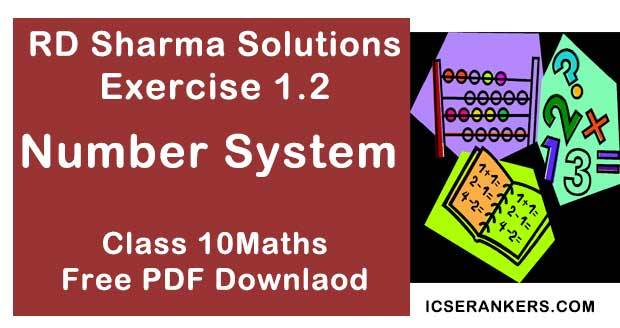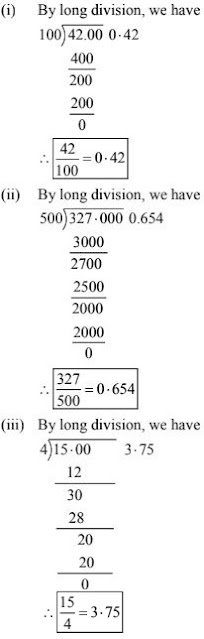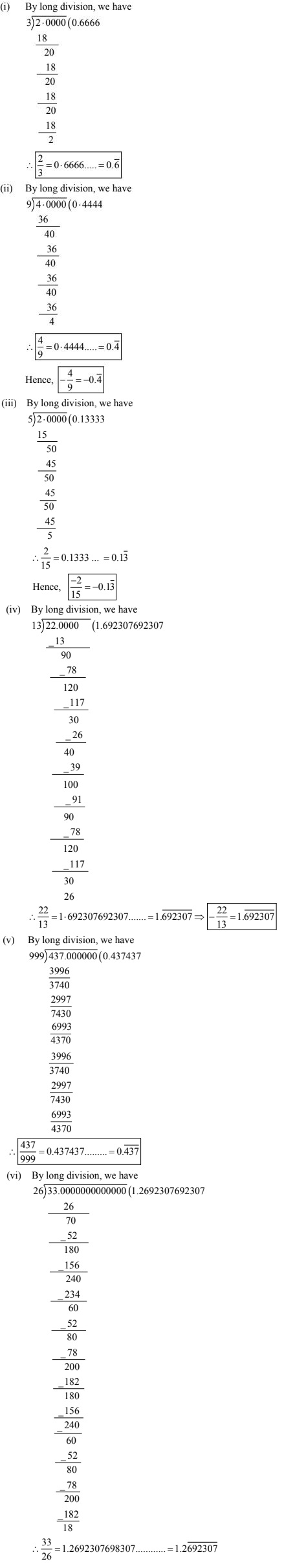# Chapter 1 Number System RD Sharma Solutions Exercise 1.2 Class 9 MathsChapter Name RD Sharma Chapter 1 Number System Exercise 1.2 Book Name RD Sharma Mathematics for Class 10 Other Exercises Exercise 1.1Exercise 1.3Exercise 1.4Exercise 1.5Exercise 1.6 Related Study NCERT Solutions for Class 10 Maths

### Exercise 1.2 Solutions

Express the following rational numbers as decimals :

1. (i) 42/100
(ii) 327/500
(iii) 15/4

Solution2. (i) 2/3
(ii) -4/9
(iii) -2/15
(iv) -22/13
(v) 437/999

Solution3. Look at several examples of rational numbers in the form p/q (q ≠ 0), where p and q are integers with no common factors other than 1 and having terminating decimal representations. Can you guess what property q must satisfy ?

Solution

A rational number p/q is a terminating decimal only, when prime factors of q are q and 5 only. Therefore, p/q is a terminating decimal only, when prime factorization of q must have only powers of 2 or 5 or both.